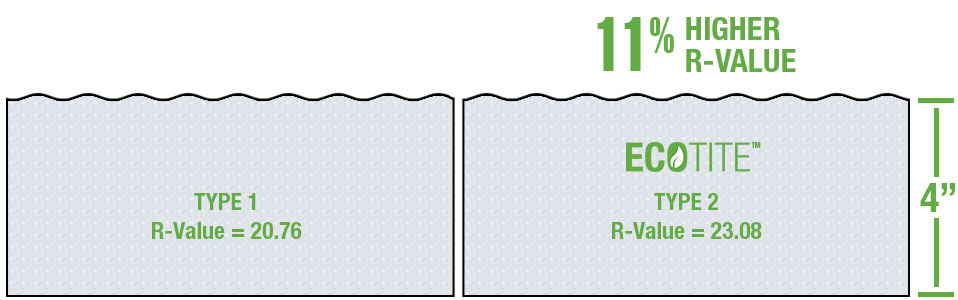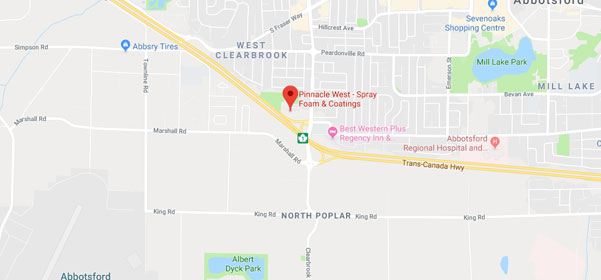What is LTTR?

Long Term Thermal Resistance

All spray foam insulation manufactured with the intent to retain a blowing agent, other than air, needs to be tested for long-term thermal resistance (LTTR) in accordance with CAN/ULC-S770. This procedure defines the long-term thermal resistance (LTTR) of a foam product as the value is measured after 5 years.

Why Measure LTTR At 5 Years?

R-values will deteriorate over time. Closed cell foam insulation is blown with gases which have a lower conductivity than air, these gases are trapped in the cells of the foam adding to the R-value. However, over time a small amount of the cells close to the surface of the foam are infiltrated by air, thus reducing the effective R-value of the product. Most of this deterioration will occur in the first year or two which is why we measure LTTR at 5 years, the decrease in R-Value after five years is minimal.

RSI vs R-Value

In Canada R-values are given in SI units (International System of Units), or the abbreviation "RSI" for the SI definition. RSI ratings are always tested on a 50mm thick sample.

Metric to US units

Values may be converted from RSI units to US units: RSI × 5.678 = R-value (US)

Or converted from US units to RSI units: R-value × 0.1761 = RSI (SI)

Type 1 vs Type 2 Foams

Type 1 foam must have an RSI rating of 1.8 or greater, whereas a Type 2 foam requires an RSI rating greater than 2.0. Quite simply, an RSI of 1.8 requires slightly more foam to achieve an R-20 value than does a foam with a 2.0 RSI rating. RSI ratings are always tested on a 50mm thick sample.

Sample calculation - TYPE 1 Foam

• So you take the RSI rating of 1.8 at a 50mm thickness and multiply it by 5.678 to get its R-value which equals 10.22.
• We can then divide 10.22 by 2 which will give an R-Value of 5.11 at 25mm.
• This can then be converted to R-Value per inch by multiplying 5.11 by 1.016 which gives us an R-Value of 5.19 per inch.
• If you require a R-20 installation you will require 97.7mm (3.85 inches) of Type 1 foam.

Sample calculation - TYPE 2 Foam

• So you take the RSI rating of 2.0 at a 50mm thickness and multiply it by 5.678 to get its R-value which equals 11.36.
• We can then divide 11.36 by 2 which will give an R-Value of 5.68 at 25mm.
• This can then be converted to R-Value per inch by multiplying 5.68 by 1.016 which gives us an R-Value of 5.77 per inch.
• If you require a R-20 installation you will require 88.1mm (3.47 inches) of Type 2 foam.

Why is this Important?

What this means is that if you are comparing quotes that discuss foam thickness, a Type 1 installation of 4 inches of spray foam is going to only provide a long term R-Value of 20.76, while a Type 2 installation of 4 inches of spray foam will provide a long term R-Value of 23.08. Don’t be fooled by a depth only comparison.ECOTITE™ is proud to deliver Type 2 CAN/ULC certified products to the Canadian spray foam insulation industry.31897 Mercantile Way
Abbotsford, BC, Canada V2T 4C3

Telephone:
Toll Free: 1-800-901-0088
International: +1-604-854-5968
Fax: 604-854-5918

Hours of Operation:
Mon to Fri: 8:00am to 4:30pm
Pacific Time

Email: